•研究论文
• 最近在研究椭圆曲线算法和双线性对算法，谁有对应的C代码，求大神指教。c 代码 算法
• I want to draw ellipses, hyperbolas in R.How can I do it using ggplot2?Let me give an examplex^2 +3xy+ 4x+ 2y+ 2y^2 = 0forx between -2 and 2y between -2 and 2解决方案Construct a data field:d y=seq(-2,...I want to draw ellipses, hyperbolas in R.How can I do it using ggplot2?Let me give an examplex^2 +3xy+ 4x+ 2y+ 2y^2 = 0forx between -2 and 2y between -2 and 2解决方案Construct a data field:d y=seq(-2,2,length=51)),z=x^2+3*x*y+4*x+2*y+2*y^2)Make a basic geom_contour plot with each level coloured differently:g1 Now get rid of the lines for all but the "0" level.  This depends on stat_contour picking zero as one of its contour levels, which might be fragile under some circumstances ... (It's not obvious to me that stat_contour() allows control of what levels are chosen, equivalent to the levels argument to contour() or contourLines() in base R -- if it did, this process would be a little bit easier.  One might submit a wishlist item to https://github.com/hadley/ggplot2/issues , if one cared enough about this ...)g1 + scale_colour_discrete(breaks="0",limits=c("0","0"),na.value=NA,guide="none")展开全文• 原标题：奇妙一招：如何用“尺规作图”作出椭圆双曲线抛物线？ 许兴华数学(图片来自万邦朝圣，特此感谢！)众所周知，在高中数学学习过程中，如果学习到“椭圆双曲线、抛物线”(简称为圆锥曲线)这一内容时，为了...
原标题：奇妙一招：如何用“尺规作图”作出椭圆双曲线抛物线？许兴华数学(图片来自万邦朝圣，特此感谢！)众所周知，在高中数学学习过程中，如果学习到“椭圆、双曲线、抛物线”(简称为圆锥曲线)这一内容时，为了加深学生对圆锥曲线三个定义的理解，教师一般都要做出这三种图形。而作出这三种圆锥曲线图形的有效方法，目前比较流行的当然是运用“几何画板”方法(这个方法，教师在课堂上可以分别演示给学生观看)，但在上课时，由于并不是每个学生都有一台电脑，所以我们教师就做不到，让人人动手来亲自画圆锥曲线。有鉴于此，今天，我们就建议各位老师给学生来一个“奇妙一招”：如何用“尺规作图”作出椭圆、双曲线和抛物线？用尺规作图来作做圆锥曲线的图形，效果是显而易见的：每个人都可以有圆规和三角板，在课堂上能做到让全班同学人人动手，这对学生深刻理解三种“圆锥曲线”的定义是有非常大的帮助的。(首先，大家须记住：“尺规作图”是指用圆规和没有刻度的直尺来作几何图形的一种方法)【一】用尺规作图作出椭圆(基本步骤和方法)1、先作出两条互相垂直的直线分别作为x轴和y轴，它们的交点为原点O，任意给出两条线段AB=2a，EF=2c(a>c>0),(此时E、F即为椭圆的两个焦点)，在x轴上依次截取OA=OB=a,OE=OF=c.于是，得到点A、B是椭圆上的两个点；2、在线段EF上任取一点C，以E为圆心、AC为半径画圆C，以F为圆心、BC为半径画圆F，两圆相交于G、H两点，则又得到椭圆上的两点G,H(如下图1)。3、在线段EF上任取另一点J，以E为圆心、AJ为半径画圆E，以F为圆心、BJ为半径画圆F，两圆相交于I、K两点，则又得到椭圆上的两点I,K(如下图2)。4.在线段EF上，模仿步骤3，不断地改变J点的位置，依照上面方法可画出椭圆上其它的点L、M，......，(如图3，图4)5.用光滑的曲线依次把以上得到的点A、G、I、L、H、K、M，......连结起来,我们就得到了所要作的椭圆的图形(如图4).证明从略，请读者自己证明一下。【二】用尺规作图作出双曲线(基本步骤和方法)1、先作出两条互相垂直的直线分别作为x轴和y轴，它们的交点为原点O，任意给出两条线段AB=2a，EF=2c(c>a>0),在x轴上依次截取OA=OB=a,OE=OF=c.(其实，E、F即为两个焦点)。于是，得到点A、B是双曲线上的两个点；2、以E为圆心、2a为半径画圆E，过点E任意作一条直线CD交圆E于一点D，连结DF，作DF的垂直平分线交直线DE于C点，则又得到双曲线上的一点C(如下图5)；3.模仿上面步骤2，不断地改变D点的位置为D1，D2，D3，......，依照上面方法可画出双曲线上其它的点H、L，......，(如图6，图7)4.用光滑的曲线依次把以上得到的点A、G、I、L、H、K、M，......连结起来,我们就得到了所要作的双曲线左支的图形(如图8).5.完全类似于以上的步骤和方法可画出双曲线的右支(如图8).证明从略，请读者自己证明一下。【三】用尺规作图作出抛物线(基本步骤和方法)1、由抛物线的定义知，抛物线上任意一点B到定点F和定直线L的距离相等。我们先作出一个定点F和一条定直线L(定点F不在定直线L上)，过点F作L的垂线，垂足为A1，再取线段FA1的中点B1，于是，得到点B1是抛物线上的一个点；2、在直线L上任意取一个异于点A1的点A2，连结FA2，作线段FA2的垂直平分线交过A2而平行于x轴的直线于点B2，连结FB2，则又得到抛物线上的一点B2(如上图9)；3.模仿上面步骤2，不断地改变A2点的位置为A3，A4，A5，......，依照上面方法可画出抛物线上其它的点B3、B4，B5......，(如图9)4.用光滑的曲线依次把以上得到的点B1、B2、B3、B4、B5、B6、......连结起来,我们就得到了所要作的抛物线的图形(如图9).证明从略，请读者自己证明一下。【关于“许兴华数学”】本公众号是南宁三中许兴华老师的微信公众号(许兴华老师是中学高级教师，南宁市学科带头人)，曾荣获得2016年数学文化杂志社主办的携手北京大学数学文化节“全国最红数学公众号”评选全国第一名。非常欢迎您关注微信公众号“许兴华数学”，获取更多的数学知识、数学方法和解题技巧！在不久的将来，我们相信，您将会深切体会到：喜爱数学，如虎添翼！精通数学，天下无敌！返回搜狐，查看更多责任编辑：
展开全文• 一、圆 圆 例 ...二、椭圆 ...椭圆的标椎方程：（右侧常数项为1） ...椭圆的面积：S = πab ...双曲线的标准方程： （右侧常数项为1） x2的系数为正，在x轴找（-a，0）（a，0） y2的系数为正，在y轴找（0，b）（0，
一、圆

圆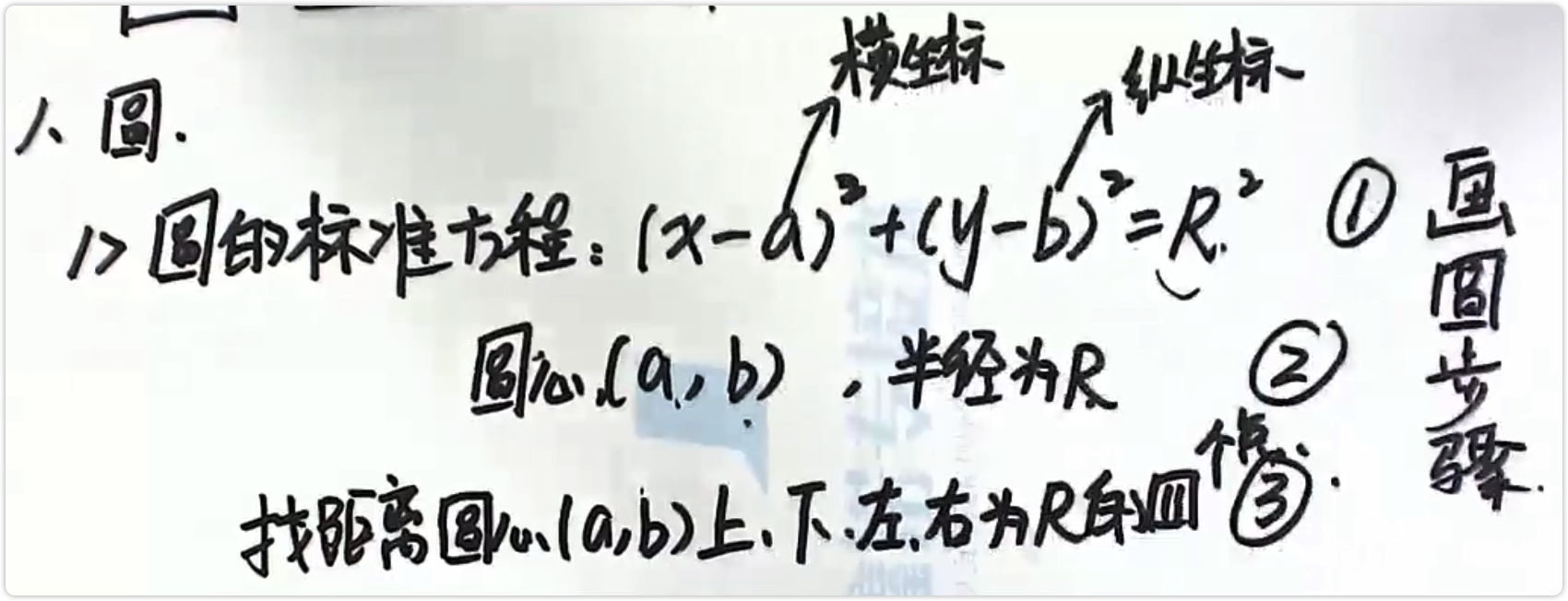例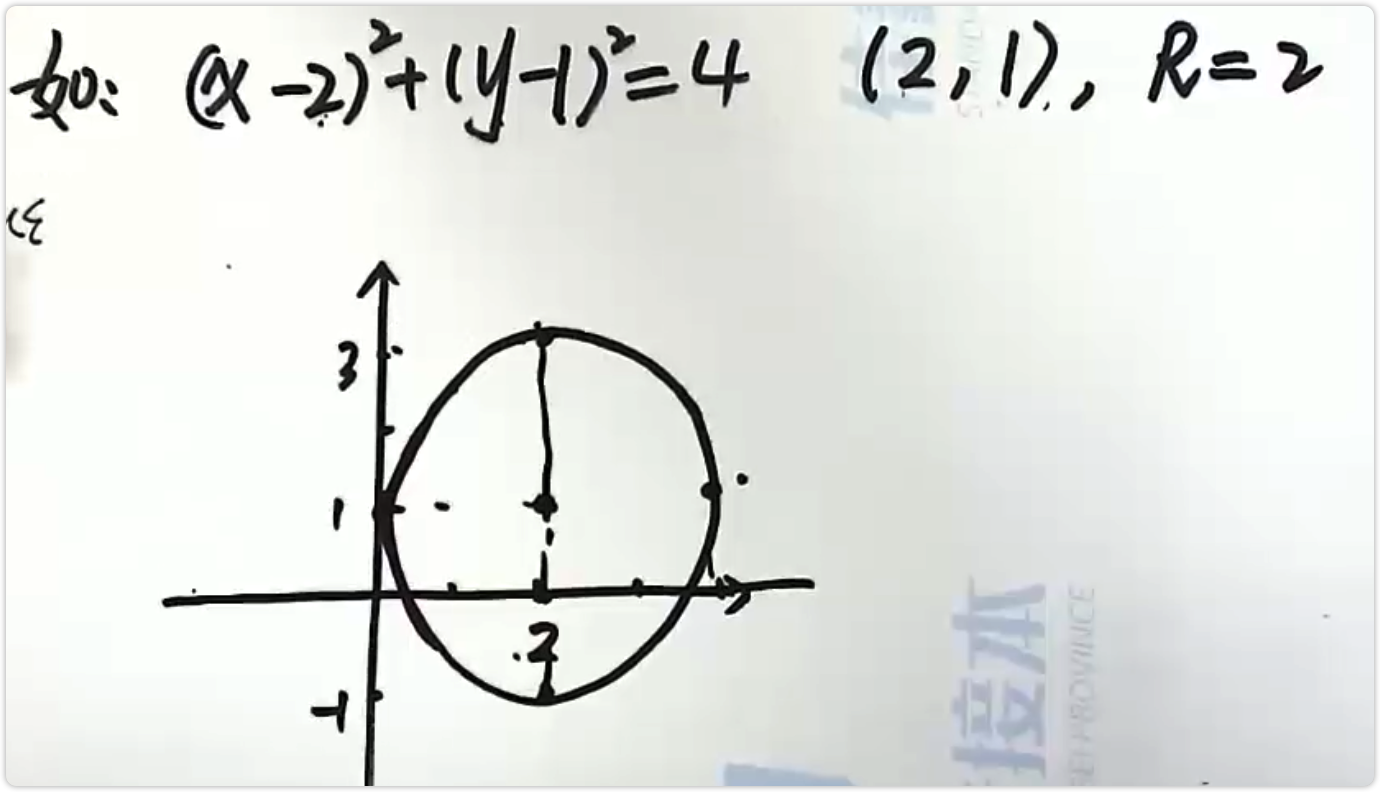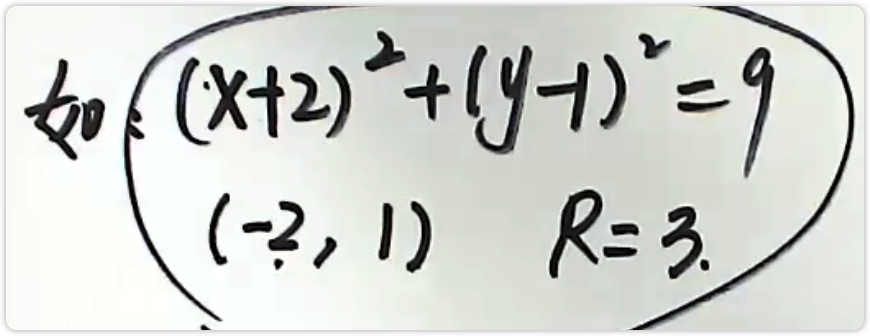圆的一般式方程：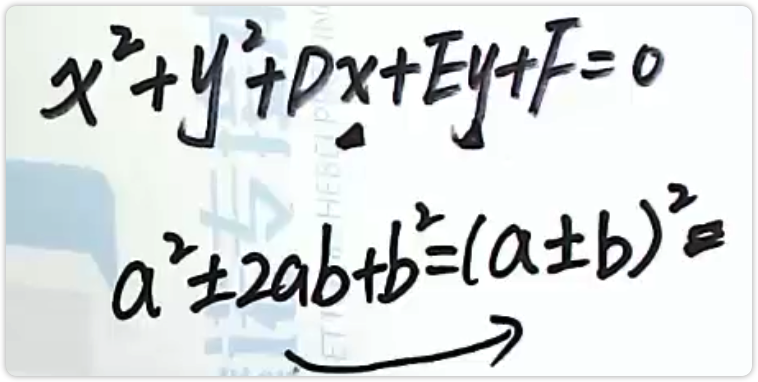例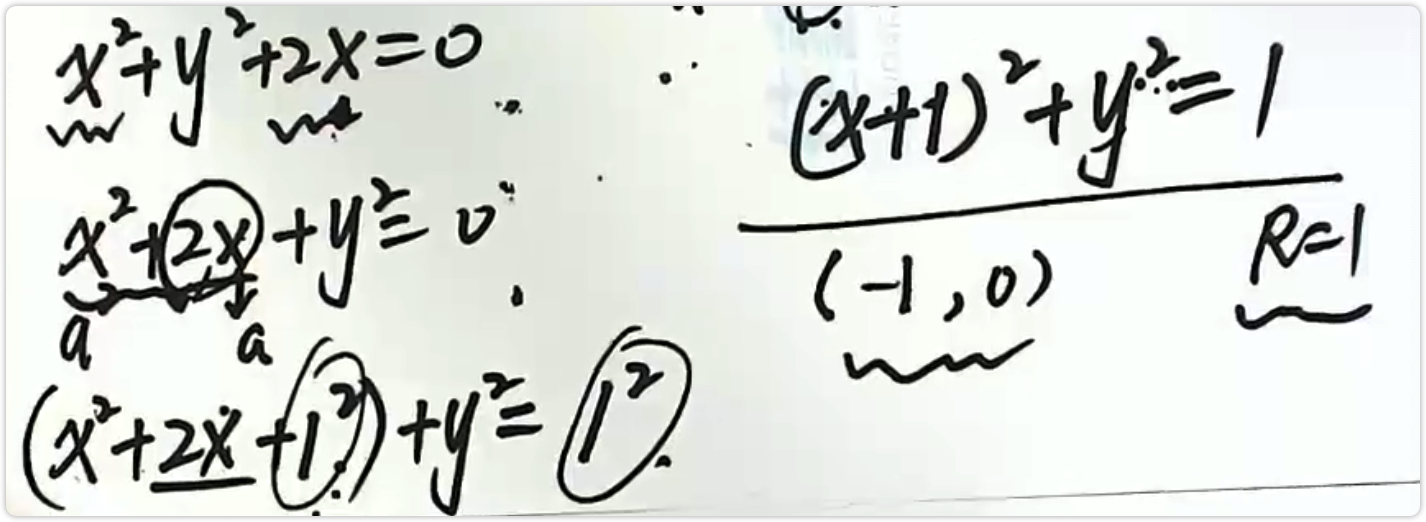圆的面积：S = πR2
圆的周长：C = 2πR

二、椭圆

椭圆的标椎方程：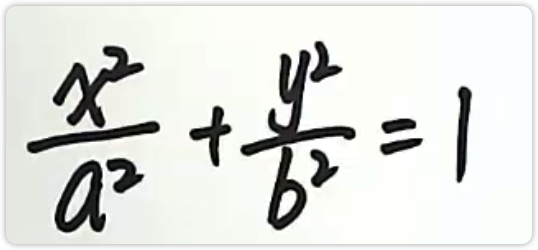（右侧常数项为1）

画图流程：

化为标准方程

找到a，b

在x轴找（-a，0）（a，0）
在y轴找（0，b）（0，-b）

例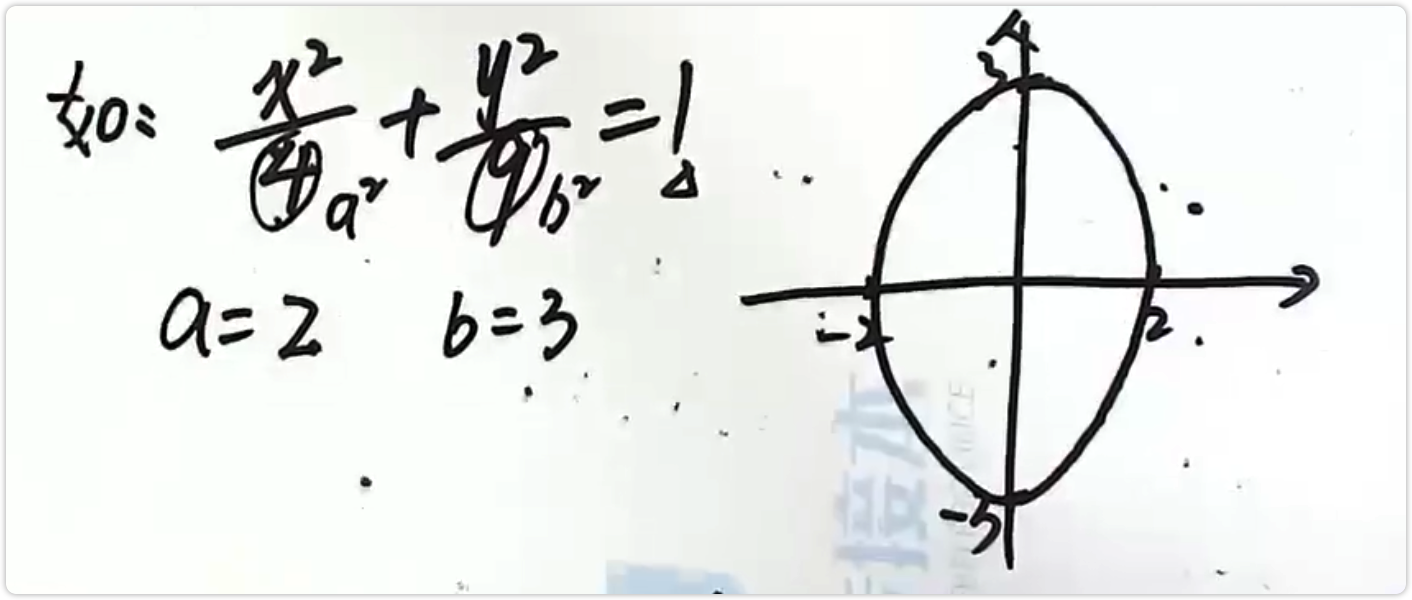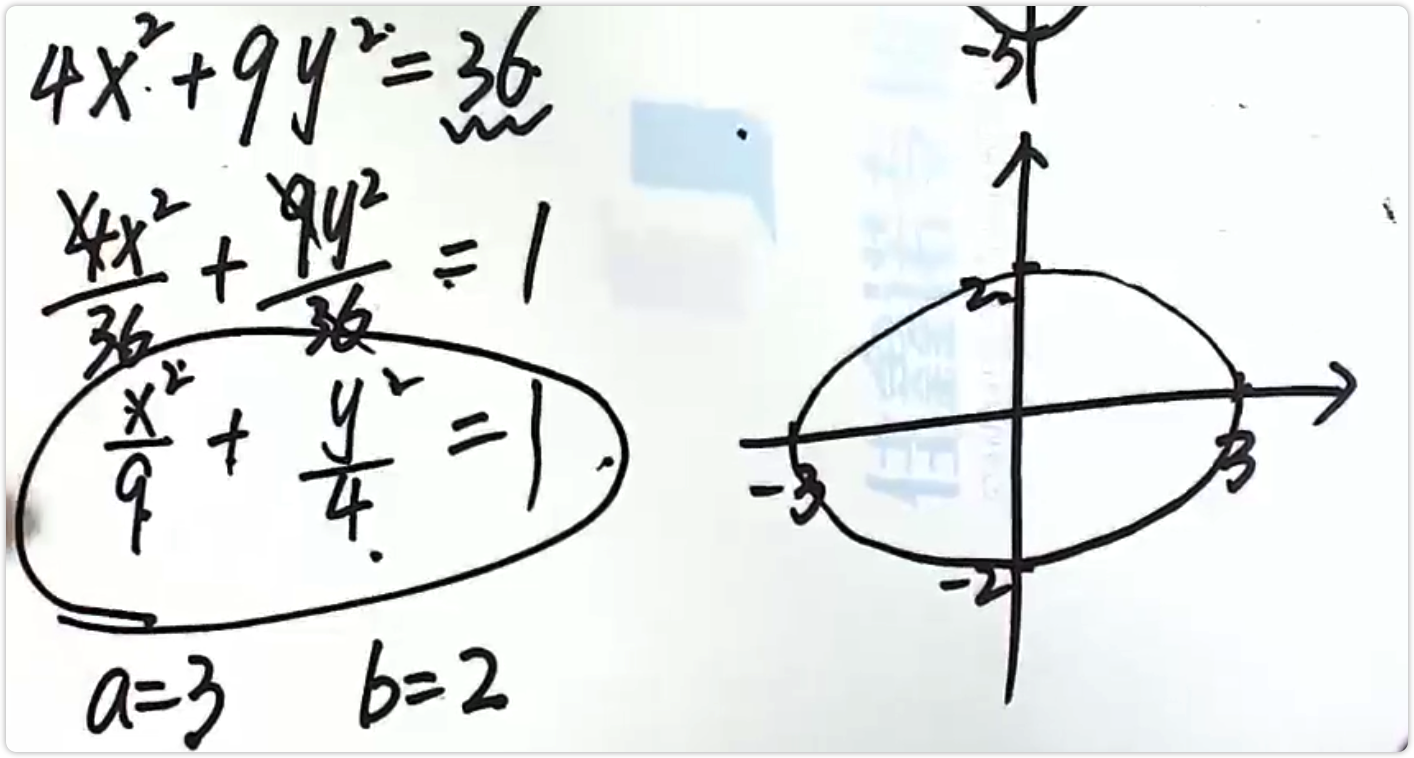椭圆的面积：S = πab

三、双曲线

双曲线的标准方程：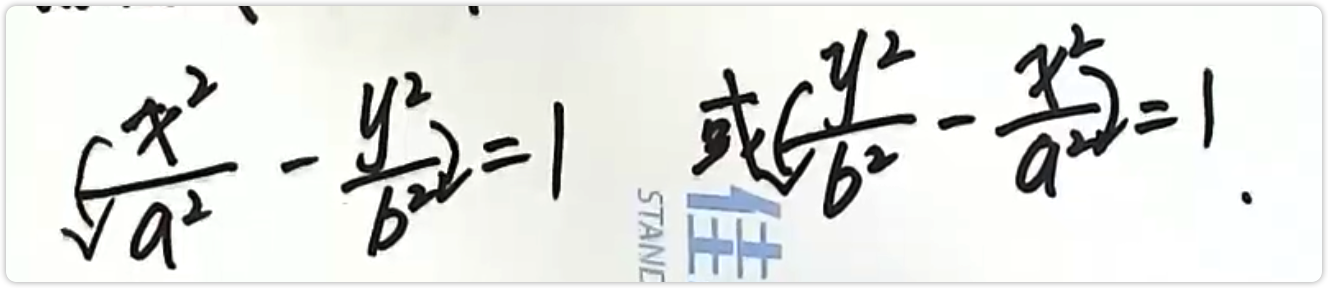（右侧常数项为1）

x2的系数为正，在x轴找（-a，0）（a，0）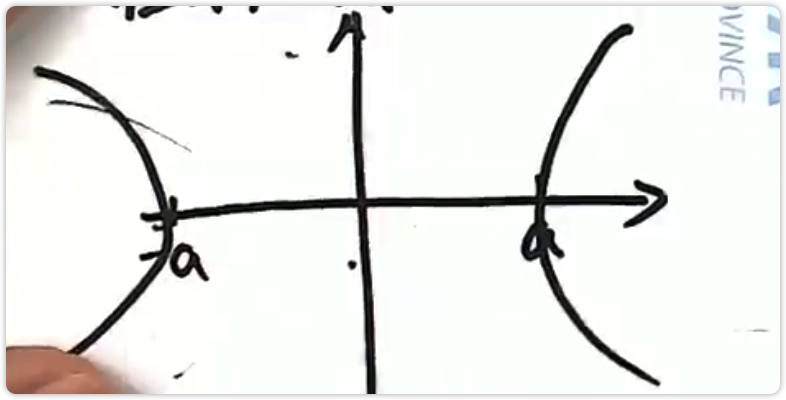y2的系数为正，在y轴找（0，b）（0，-b）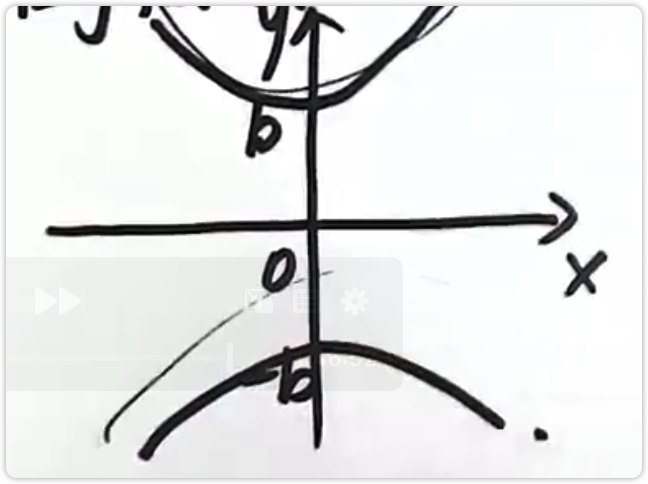总结：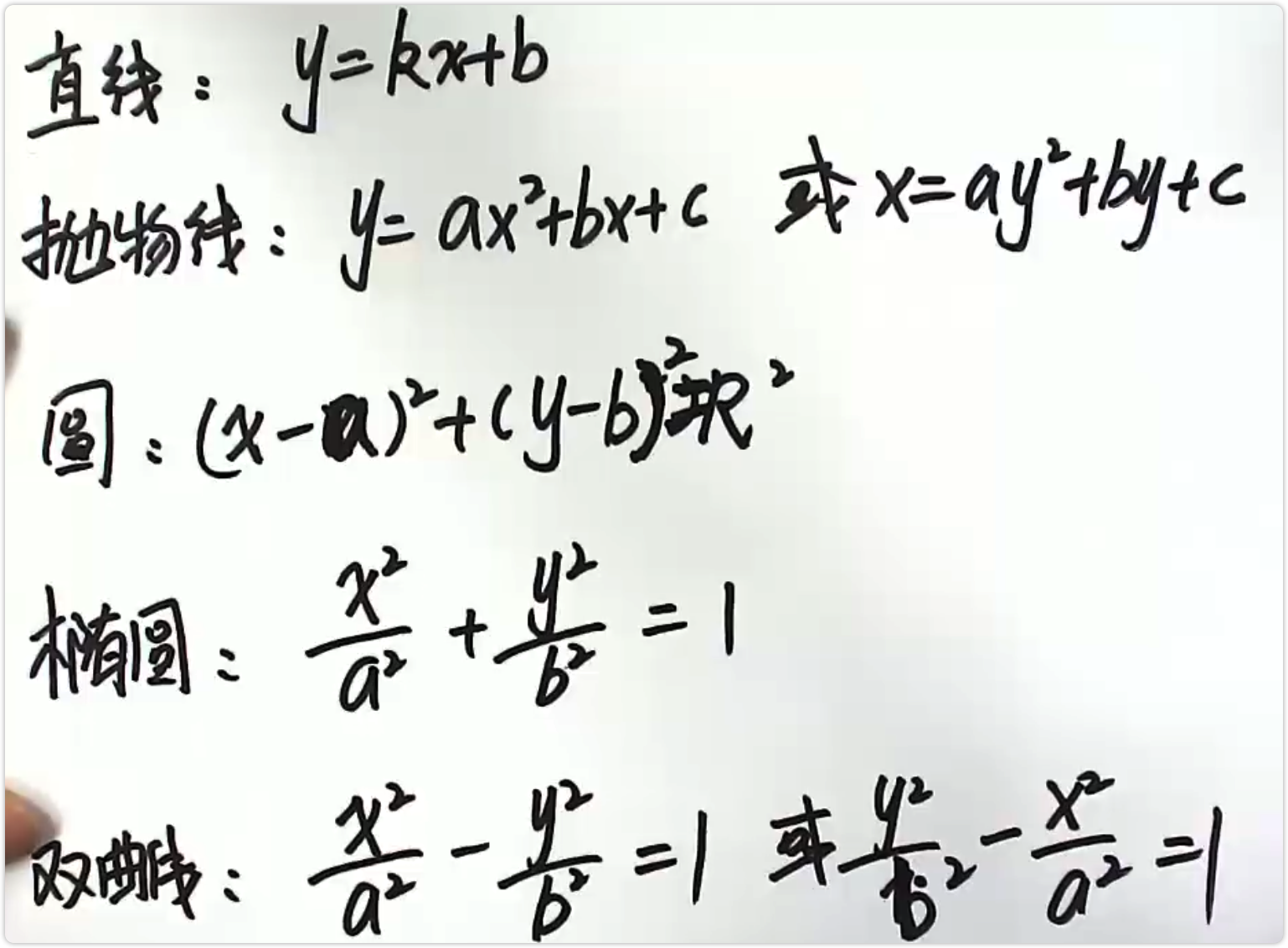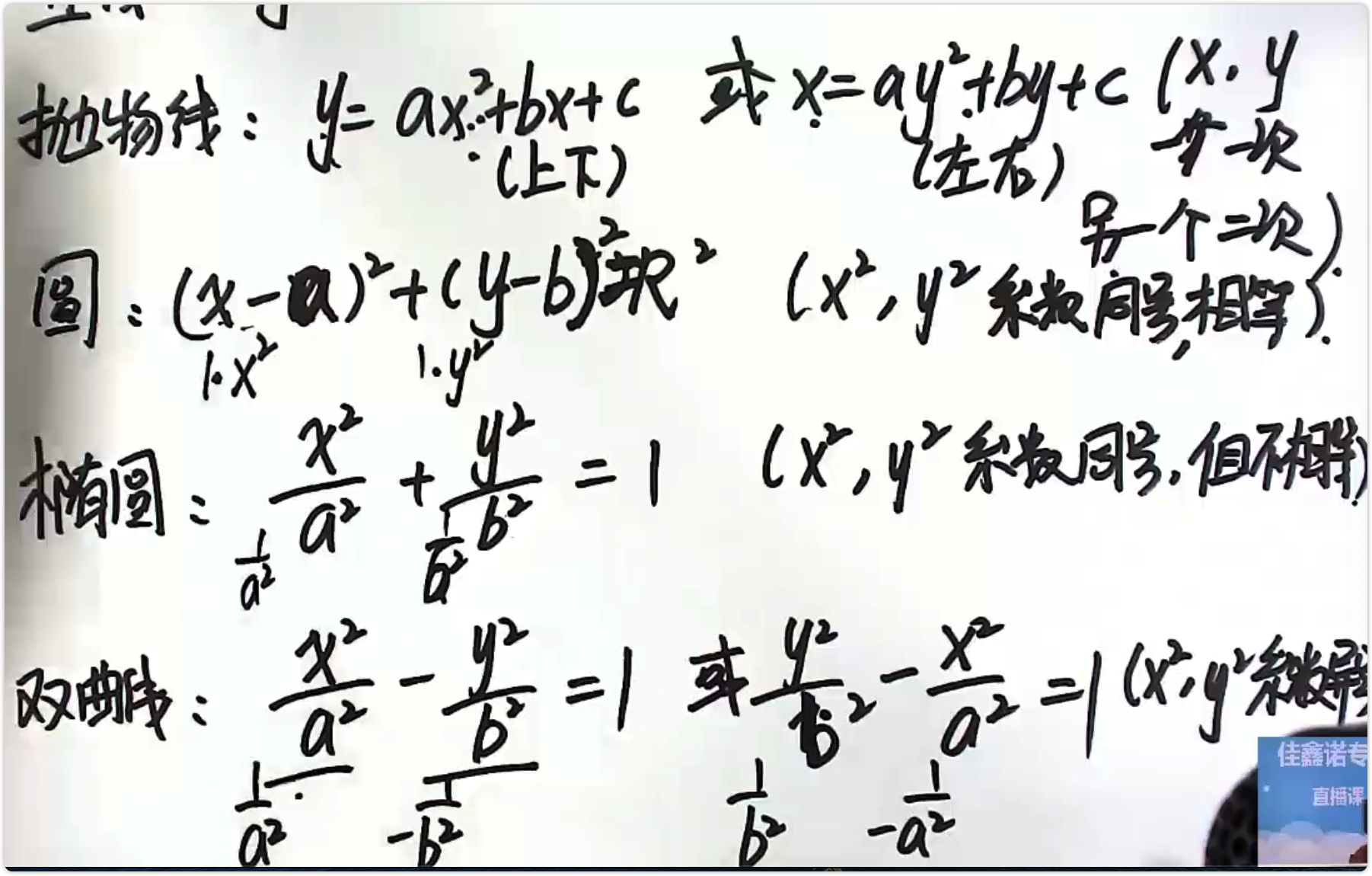展开全文• RFID技术在被广泛应用的同时，各种针对RFID标签与读写器间身份...以椭圆曲线加密体制和零知识身份证明为基础，提出一种标签与读写器双向身份认证的协议，提出一种动态标签ID的方式，更好地解决了嗅探、跟踪攻击等问题。
• 椭圆曲线密码学（ECC，Elliptic Curve Cryptogphay）是一种流行的非对称加密算法，其背后的数学原理，是椭圆曲线上的离散对数难题。我们还知道，ECC 的私钥，本质是一个整数，其对应的公钥，是椭圆曲线上的一个点。 ...

https://aaron67.cc/2020/09/30/ecdsa/

在文章非对称加密和签名认证中，我们介绍了双钥系统的两种应用场景：

加密解密时，公钥用于加密，私钥用于解密
身份认证时，私钥用于签名，公钥用于验证

椭圆曲线密码学（ECC，Elliptic Curve Cryptogphay）是一种流行的非对称加密算法，其背后的数学原理，是椭圆曲线上的离散对数难题。我们还知道，ECC 的私钥，本质是一个整数，其对应的公钥，是椭圆曲线上的一个点。
在将 ECC 作为双钥系统使用时，针对不同的应用场景，会涉及到不同的算法。常见的有

在加密和解密时使用的椭圆曲线集成加密框架（ECIES，Elliptic Curve Integrated Encryption Schema）
用于协商和交换公共密钥的椭圆曲线 Diffie-Hellman 密钥交换算法（ECDH，Elliptic Curve Diffie-Hellman Key Exchange）
用于生成和验证数字签名的椭圆曲线数字签名算法（ECDSA，Elliptic Curve Digital Signature Algorithm）

本文将介绍并实现 ECDSA 的相关内容。
签名
用私钥 $a$ 对消息 $m$ 签名，得到的结果是两个整数 $(r, s)$ ，计算过程如下。

随机生成临时私钥 $k$，并计算其对应的公钥 $K = k \cdot G = (x_K, y_K)$
计算 $r = x_K \bmod{n}$，若 $r$ 为 0，则回到第一步
计算消息 $m$ 的哈希 $e = hash(m)$，并将 $e$ 的二进制序列转成一个整数
计算 $s = k^{-1}(e + ra) \bmod{n}$，若 $s$ 为 0，则回到第一步
得到签名 $(r, s)$

import hashlib

def sha256(payload: bytes) -> bytes:
return hashlib.sha256(payload).digest()

def double_sha256(payload: bytes) -> bytes:
return sha256(sha256(payload))

import random

def hash_to_int(message: bytes) -> int:
"""Calculate the bitcoin double-sha256 hash of the message, return as an integer"""
h = double_sha256(message)
return int.from_bytes(h, byteorder='big')

def sign(private_key: int, message: bytes) -> tuple:
"""Create ECDSA signature (r, s)"""
e = hash_to_int(message)
r, s = 0, 0
while not r or not s:
k = random.randrange(1, curve.n)
k_x, _ = scalar_multiply(k, curve.g)
r = k_x % curve.n
s = ((e + r * private_key) * modular_multiplicative_inverse(k, curve.n)) % curve.n
return r, s

如果每次签名都使用相同的 $k$，当知道了消息 $e_1$、$e_2$ 和签名 $(r_1, s_1)$、$(r_2, s_2)$ 时，有

$r_1 = r_2$，因为 $k$ 相同
根据 $s$ 的定义，有 $s_1 - s_2 = k^{-1}(e_1 - e_2) \bmod{n}$
将上式两边同时乘以 $k$ 再除以 $(s_1 - s_2)$，有 $k = (s_1 - s_2)^{-1}(e_1 - e_2) \bmod{n}$

这样就得到了 $k$，再根据 $s = k^{-1}(e + ra) \bmod{n}$，从而反推出 $a = r^{-1}(sk - e) \bmod{n}$。类似的攻击方法在 $k$ 值可预测时也能使用。
所以请务必注意，每次签名时使用的 $k$，都需要保证绝对私密且生成时足够随机，以保证私钥 $a$ 的安全。
验签
用公钥 $A$ 和消息 $m$ 验证签名 $(r, s)$，过程如下。

计算消息 $m$ 的哈希 $e = hash(m)$，并将 $e$ 的二进制序列转成一个整数
计算整数 $u_1 = s^{-1}e \bmod{n}$
计算整数 $u_2 = s^{-1}r \bmod{n}$
计算点 $P = u_1 \cdot G + u_2 \cdot A = (x_P, y_P)$
当且仅当 $r = x_P \bmod{n}$ 时，验签成功

def verify_signature(public_key: tuple, message: bytes, signature: tuple) -> bool:
"""Verify signature with public key and message"""
e = hash_to_int(message)
r, s = signature
w = modular_multiplicative_inverse(s, curve.n)
u1 = (w * e) % curve.n
u2 = (w * r) % curve.n
x, _ = add(scalar_multiply(u1, curve.g), scalar_multiply(u2, public_key))
return r == (x % curve.n)

证明
在开始之前，需要先明确一点：椭圆曲线上点的加法运算，符合交换律和结合律。
所以有 $aG + bG = (a + b) \cdot G$，因为
$aG + bG = \underbrace{G + G + ... + G}\_{a\ 个} + \underbrace{G + G + ... + G}\_{b\ 个} = \underbrace{G + G + ... + G}\_{(a + b)\ 个} = (a + b) \cdot G$
进一步的，有 $a \cdot bG = ab \cdot G$，因为
$a \cdot bG = \underbrace{bG + bG + ... + bG}\_{a\ 个} = \underbrace{(G + ... + G) + ... + (G + ... + G)}\_{a\ 个} = \underbrace{G + G + ... + G}\_{ab\ 个} = ab \cdot G$
让我们借助上面这两个推论，通过公式变换，证明验签过程的正确性。
已知 $A = aG$，则
$P = u_1 \cdot G + u_2 \cdot A = u_1 \cdot G + u_2 \cdot aG = (u_1 + u_2a) \cdot G$
带入 $u_1$ 和 $u_2$ 的定义，则
$P = (u_1 + u_2a) \cdot G = (s^{-1}e + s^{-1}ra) \cdot G = s^{-1}(e + ra) \cdot G$
请注意，这里我们忽略了 $u_1$ 和 $u_2$ 定义中的模 $n$ 运算，这不会有任何问题，因为 $a \cdot G = (a \bmod{n}) \cdot G$。如果你想知道为什么，可以搜索关键字“循环子群”阅读更多资料。
我们还知道 $s = k^{-1}(e + ra) \bmod{n}$，将等式两边同时乘以 $k$ 再除以 $s$，就会得到
$k = s^{-1}(e + ra) \bmod{n}$
也就是说，如果 $(r, s)$ 正确，验签时计算出的点 $P$ 就是签名时临时私钥 $k$ 对应的公钥。
$P = s^{-1}(e + ra) \cdot G = k \cdot G = (x_P, y_P)$
根据 $r$ 的定义，即当且仅当 $r = x_P \bmod{n}$ 时，验签成功。
验证
我们可以写一个简单的例子来测试代码。
if __name__ == '__main__':
# 私钥
priv_key = 0xf97c89aaacf0cd2e47ddbacc97dae1f88bec49106ac37716c451dcdd008a4b62
# 公钥
pub_key = scalar_multiply(priv_key, curve.g)
# 要签名的消息
plain_text = '你好世界'
digest = sha256(plain_text.encode('utf-8'))
# 签名
sig_r, sig_s = sign(priv_key, digest)
print(' r =', sig_r)
print(' s =', sig_s)
# 验证签名 (r, s)
print(verify_signature(pub_key, digest, (sig_r, sig_s)))

运行结果为
r = 114587593887127314608220924841831336233967095853165151956820984900193959037698
s = 24000727837347392504013031837120627225728348681623127776947626422811445180558
True

输出符合预期。
签名的“对称性”
对 ECDSA 签名 $(r, s)$，在验证时如果使用 $(r, -s \bmod{n})$，则也能验签成功。
if __name__ == '__main__':
#
# ...
#
# 验证签名 (r, -s)
negative_s = -sig_s % curve.n
print('-s =', negative_s)
print(verify_signature(pub_key, digest, (sig_r, negative_s)))

运行结果为
 r = 106466997694091524629965845090867478458136818253940782993316021692670806749258
s = 62904381853960967395432938678025872957596216909471919761592805395928247608025
True
-s = 52887707383355228028138046330662034895241347369602984621012357745589913886312
True

这个“特性”本文暂不展开介绍，直接把结论放在这里，以保证整体内容的完整性。
完整代码
sign.py
参考

Elliptic Curve Cryptography: a gentle introduction 原文 译文
Elliptic Curve Cryptography: finite fields and discrete logarithms 原文 译文
Elliptic Curve Cryptography: ECDH and ECDSA 原文 译文
GitHub andreacorbellini/ecc
ECDSA 详解
椭圆曲线加密算法
Digital Signatures (Signing & Verifying)


展开全文比特币 bitcoin
• 展开全部几何画板作为专业的绘图工具，用它绘制椭圆不仅可以使图形画32313133353236313431303231363533e4b893e5b19e31333361326238得更准确，还能很好地显示椭圆的一些性质，具体步骤如下：1.构造线段与中点。选择...
• java借助bouncycastle库实现ECC双向加密解密算法Utils工具包。
• JPBC库是一个功能很强大的数学库，用于生成椭圆曲线，双线性等，但网上参考资料很少，重复度极高，该分栏用于安装，JPBC参数解释，生成椭圆曲线群，整数群（不用双线性性质），双线性映射教学。内容原创，禁止任何...java eclipse
• 乐学数韵(ID/抖音：Vlxsy8 视频号/B站：乐学数...高考数学：巧用压缩变换来求解一些椭圆问题4.2021年考情教学——椭圆、抛物线、双曲线5.精品微课：极坐标与参数方程系列(2)圆的参数方程+抛物线参数方程+椭圆和双曲...
• 椭圆曲线 Wikipedia https://en.wikipedia.org/wiki/Elliptic_curve_cryptography   http://www.isg.rhul.ac.uk/~sdg/ecc.html   Elliptic Curve Cryptography   ellipticnews ...双线性对
• 椭圆双曲线、抛物线的标准方程与几何性质 http://dy.163.com/v2/article/detail/DGSH79TK0521JEM8.html 椭圆 双曲线 抛物线 定义 1．到两定点F1,F2的距离之和为定值2a(2a>|F1F2|)的点的轨迹 ...
• 基于miracl库实现椭圆曲线算法，包括双线性对的实现，塔式扩张等
• 椭圆双曲线、抛物线 定义和概念有点多，稍微整理一下 名称/性质 标准方程 范围 对称性 顶点 离心率 渐近线 椭圆 $$\frac{x^2}{a^2} + \frac{y^2}{b^2} = 1, (a>b>0)$$ \(-a \leqslant x \leqslant a,...
• 在本文中，我们提出了一种基于自认证公钥系统和椭圆曲线密码学的WANET用户认证方案。 使用所提出的方案，可以实现有效的双向用户认证和安全的会话密钥协议。 安全分析表明，我们提出的方案可以抵抗常见的已知攻击。...
• 对超椭圆曲线上一类非退化的Ate对变种进行研究,使得计算双线性对的Miller算法的循环次数显著减少。通过对此类双线性对与改进Tate对及Ate对的关系的一系列证明,验证了此类双线性对的非退化性;基于广义的Ate对和...
• 在jPBC中，双线性群的使用都是通过叫做Pairing的对象来实现的。...Type A1曲线需要二个参数：numPrime是阶数N中有几个质数因子；qBit是每个质数因子的比特长度。注意，Type A1涉及到的阶数很大，其...
• 基于椭圆曲线密码体制的遥感图像加密算法研究，时向勇，李先华，在介绍Montgomery型椭圆曲线体制的基础上，结合Montgomery型椭圆曲线标量乘的快速性和基于证书的ECDH椭圆曲线密钥交换协议的双向性等特性蒙哥马利
• \[\begin{cases}\cfrac{x^2}{a^2} + \cfrac{y^2}{b^2} = 1\\y = kx + m\end{cases}\\(a^2k^2+b^2)x^2+2kma^2x+a^2m^2-a^2b^2=0\\\Delta = 4a^2b^2(A-m^2)=4a^2b^2(a^2k^2+b^2-m^2)\\\begin{cases}x_1+x_2 =\cfrac{-2...
• 1，这n个点分布在椭圆曲线方程上，其中为点与拟合的期望方程的偏差。 在xy坐标系中，椭圆方程的基本形式是：。 证明如下： 圆锥曲线上的每一点与曲线外的某点的距离，与其到某直线l: 的距离之比为...behavior matlab 图像处理 url function
• 椭圆曲线代理签名相关论文计算机技术的高速发展为人类提供了高度的自动化和现代化，网络的迅猛发展为人 们提供了便捷、快速的信息交流方式，使我们人类社会迅速进入了信息化时代。互联网 的发展和壮大使得人们...
• 例1：如图，直线与双曲线的左、右两支分别交于两点，与双曲线的右准线相交于点，为右焦点，若，又，则实数的取值为. 解法一，第二定义： 由定义得，由相似得.解法二，坐标：，，故，故还行，你还我第二定义.例2：...
• 圆：相切相交写反了 椭圆双曲线：抛物线:很方便 写的时候马虎可能会有些小瑕疵圆的相切相交相离公式
• \[ \begin{cases} \cfrac{x^2}{a^2} + \cfrac{y^2}{b^2} = 1\\ y = kx + m \end{cases}\\ (a^2k^2+b^2)x^2+2kma^2x+a^2m^2-a^2b^2=0\\ \Delta = 4a^2b^2(A-m^2)=4a^2b^2(a^2k^2+b^2-m^2)\\ \begin{cases} ......